International Tables for Crystallography (2006). Vol. D, ch. 1.3, pp. 72-98https://doi.org/10.1107/97809553602060000630

## Contents

• 1.3. Elastic properties  (pp. 72-98)
• 1.3.1. Strain tensor  (pp. 72-76) | html | pdf |
• 1.3.1.1. Introduction, the notion of strain field  (p. 72) | html | pdf |
• 1.3.1.2. Homogeneous deformation  (pp. 72-74) | html | pdf |
• 1.3.1.2.1. Fundamental property of the homogeneous deformation  (p. 72) | html | pdf |
• 1.3.1.2.2. Spontaneous strain  (p. 72) | html | pdf |
• 1.3.1.2.3. Cubic dilatation  (pp. 72-73) | html | pdf |
• 1.3.1.2.4. Expression of any homogeneous deformation as the product of a pure rotation and a pure deformation  (p. 73) | html | pdf |
• 1.3.1.2.5. Quadric of elongations  (pp. 73-74) | html | pdf |
• 1.3.1.3. Arbitrary but small deformations  (pp. 74-75) | html | pdf |
• 1.3.1.3.1. Definition of the strain tensor  (pp. 74-75) | html | pdf |
• 1.3.1.3.2. Geometrical interpretation of the coefficients of the strain tensor  (p. 75) | html | pdf |
• 1.3.1.4. Particular components of the deformation  (pp. 75-76) | html | pdf |
• 1.3.1.4.1. Simple elongation  (p. 75) | html | pdf |
• 1.3.1.4.2. Pure shear  (p. 76) | html | pdf |
• 1.3.1.4.3. Simple shear  (p. 76) | html | pdf |
• 1.3.2. Stress tensor  (pp. 76-80) | html | pdf |
• 1.3.2.1. General conditions of equilibrium of a solid  (p. 76) | html | pdf |
• 1.3.2.2. Definition of the stress tensor  (pp. 76-77) | html | pdf |
• 1.3.2.3. Condition of continuity  (p. 77) | html | pdf |
• 1.3.2.4. Symmetry of the stress tensor  (pp. 77-78) | html | pdf |
• 1.3.2.5. Voigt's notation – interpretation of the components of the stress tensor  (p. 78) | html | pdf |
• 1.3.2.5.1. Voigt's notation, reduced form of the stress tensor  (p. 78) | html | pdf |
• 1.3.2.5.2. Interpretation of the components of the stress tensor – special forms of the stress tensor  (p. 78) | html | pdf |
• 1.3.2.6. Boundary conditions  (pp. 78-79) | html | pdf |
• 1.3.2.7. Local properties of the stress tensor  (p. 79) | html | pdf |
• 1.3.2.8. Energy density in a deformed medium  (pp. 79-80) | html | pdf |
• 1.3.3. Linear elasticity  (pp. 80-85) | html | pdf |
• 1.3.3.1. Hooke's law  (pp. 80-81) | html | pdf |
• 1.3.3.2. Elastic constants  (pp. 81-82) | html | pdf |
• 1.3.3.2.1. Definition  (p. 81) | html | pdf |
• 1.3.3.2.2. Matrix notation – reduction of the number of independent components  (pp. 81-82) | html | pdf |
• 1.3.3.2.3. Passage from elastic compliances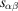to elastic stiffnesses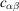(p. 82) | html | pdf |
• 1.3.3.3. Elastic strain energy  (p. 82) | html | pdf |
• 1.3.3.4. Particular elastic constants  (pp. 82-84) | html | pdf |
• 1.3.3.4.1. Volume compressibility  (pp. 82-83) | html | pdf |
• 1.3.3.4.2. Linear compressibility  (p. 83) | html | pdf |
• 1.3.3.4.3. Young's modulus, Poisson's ratio  (p. 83) | html | pdf |
• 1.3.3.4.4. Variation of Young's modulus with orientation  (pp. 83-84) | html | pdf |
• 1.3.3.5. Isotropic materials  (pp. 84-85) | html | pdf |
• 1.3.3.6. Equilibrium conditions of elasticity for isotropic media  (p. 85) | html | pdf |
• 1.3.4. Propagation of elastic waves in continuous media – dynamic elasticity  (pp. 86-89) | html | pdf |
• 1.3.4.1. Introduction  (p. 86) | html | pdf |
• 1.3.4.2. Equation of propagation of a wave in a material  (p. 86) | html | pdf |
• 1.3.4.3. Dynamic elastic stiffnesses  (p. 86) | html | pdf |
• 1.3.4.4. Polarization of the elastic waves  (pp. 86-87) | html | pdf |
• 1.3.4.5. Relation between velocity of propagation and elastic stiffnesses  (p. 87) | html | pdf |
• 1.3.4.5.1. Cubic crystals  (p. 87) | html | pdf |
• 1.3.4.5.2. Hexagonal crystals  (p. 87) | html | pdf |
• 1.3.4.5.3. Tetragonal crystals (classes,,)  (p. 87) | html | pdf |
• 1.3.4.6. Experimental determination of elastic constants  (pp. 88-89) | html | pdf |
• 1.3.4.6.1. Introduction  (p. 88) | html | pdf |
• 1.3.4.6.2. Resonance technique  (p. 88) | html | pdf |
• 1.3.4.6.3. Pulse-echo techniques  (pp. 88-89) | html | pdf |
• 1.3.5. Pressure dependence and temperature dependence of the elastic constants  (pp. 89-91) | html | pdf |
• 1.3.5.1. Introduction  (pp. 89-90) | html | pdf |
• 1.3.5.2. Temperature dependence of the elastic constants  (pp. 90-91) | html | pdf |
• 1.3.5.3. Pressure dependence of the elastic constants  (p. 91) | html | pdf |
• 1.3.6. Nonlinear elasticity  (pp. 91-94) | html | pdf |
• 1.3.6.1. Introduction  (pp. 91-92) | html | pdf |
• 1.3.6.2. Lagrangian and Eulerian description  (p. 92) | html | pdf |
• 1.3.6.3. Strain and stress tensors  (pp. 92-93) | html | pdf |
• 1.3.6.4. Second-order and higher-order elastic stiffnesses  (p. 93) | html | pdf |
• 1.3.6.5. Expansion of elastic constants for small initial stress  (p. 93) | html | pdf |
• 1.3.6.6. Elastic strain-energy density  (p. 94) | html | pdf |
• 1.3.7. Nonlinear dynamic elasticity  (pp. 94-97) | html | pdf |
• 1.3.7.1. Introduction  (p. 94) | html | pdf |
• 1.3.7.2. Equation of motion for elastic waves  (pp. 94-95) | html | pdf |
• 1.3.7.3. Wave propagation in a nonlinear elastic medium   (pp. 95-96) | html | pdf |
• 1.3.7.3.1. Isotropic media  (p. 95) | html | pdf |
• 1.3.7.3.2. Cubic media (most symmetrical groups)  (pp. 95-96) | html | pdf |
• 1.3.7.4. Harmonic generation  (p. 96) | html | pdf |
• 1.3.7.5. Small-amplitude waves in a strained medium  (pp. 96-97) | html | pdf |
• 1.3.7.6. Experimental determination of third- and higher-order elastic constants  (p. 97) | html | pdf |
• 1.3.8. Glossary  (p. 97) | html | pdf |
• References | html | pdf |
• Figures
• Fig. 1.3.1.1. Displacement vector,(p. 72) | html | pdf |
• Fig. 1.3.1.2. Elongation,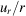(p. 73) | html | pdf |
• Fig. 1.3.1.3. Quadric of elongations  (p. 74) | html | pdf |
• Fig. 1.3.1.4. Geometrical interpretation of the components of the strain tensor  (p. 75) | html | pdf |
• Fig. 1.3.1.5. Special deformations  (p. 75) | html | pdf |
• Fig. 1.3.2.1. Definition of stress  (p. 76) | html | pdf |
• Fig. 1.3.2.2. Stress,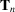, applied to the surface of an internal volume  (p. 76) | html | pdf |
• Fig. 1.3.2.3. Equilibrium of a small volume element  (p. 77) | html | pdf |
• Fig. 1.3.2.4. Symmetry of the stress tensor  (p. 78) | html | pdf |
• Fig. 1.3.2.5. Special forms of the stress tensor  (p. 79) | html | pdf |
• Fig. 1.3.2.6. Normal () and shearing () stress  (p. 79) | html | pdf |
• Fig. 1.3.2.7. The stress quadric  (p. 80) | html | pdf |
• Fig. 1.3.2.8. Determination of the energy density in a deformed medium  (p. 80) | html | pdf |
• Fig. 1.3.3.1. Bar loaded in pure tension  (p. 80) | html | pdf |
• Fig. 1.3.3.2. Schematic stress–strain curve  (p. 81) | html | pdf |
• Fig. 1.3.3.3. Spherical coordinates  (p. 84) | html | pdf |
• Fig. 1.3.3.4. Representation surface of the inverse of Young's modulus  (p. 85) | html | pdf |
• Fig. 1.3.4.1. Resonance technique: standing waves excited in a parallelepiped  (p. 89) | html | pdf |
• Fig. 1.3.4.2. Block diagram of the pulse-echo technique  (p. 89) | html | pdf |
• Fig. 1.3.5.1. Temperature dependence of the elastic stiffnesses of an aluminium single crystal  (p. 89) | html | pdf |
• Fig. 1.3.5.2. Pressure dependence of the elastic stiffness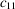of a KZnF 3 crystal  (p. 90) | html | pdf |
• Fig. 1.3.5.3. Temperature dependence of the elastic constant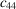in RbCdF 3 , CsCdF 3 and TlCdF 3 crystals  (p. 90) | html | pdf |
• Fig. 1.3.5.4. Temperature dependence of the elastic constantin KNiF 3   (p. 91) | html | pdf |
• Fig. 1.3.5.5. Temperature dependence of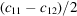in DyVO 4   (p. 91) | html | pdf |
• Fig. 1.3.5.6. Pressure dependence of the elastic constantsin TlCdF 3   (p. 91) | html | pdf |
• Tables
• Table 1.3.2.1. Stresses applied to the faces surrounding a volume element  (p. 77) | html | pdf |
• Table 1.3.3.1. Number of independent components of the elastic compliances and stiffnesses for each Laue class  (p. 82) | html | pdf |
• Table 1.3.3.2. Elastic compliances of some cubic materials in (GPa) −1 (after Landoldt-Börnstein, 1979)  (p. 83) | html | pdf |
• Table 1.3.3.3. Relations between elastic coefficients in isotropic media  (p. 86) | html | pdf |
• Table 1.3.4.1. Velocity of propagation when the wavevector is parallel to  (cubic crystals)  (p. 87) | html | pdf |
• Table 1.3.4.2. Velocity of propagation when the wavevector is parallel to  (cubic crystals)  (p. 87) | html | pdf |
• Table 1.3.4.3. Velocity of propagation when the wavevector is parallel to  (cubic crystals)  (p. 87) | html | pdf |
• Table 1.3.4.4. Velocity of propagation when the wavevector is parallel to  (hexagonal crystals)  (p. 87) | html | pdf |
• Table 1.3.4.5. Velocity of propagation when the wavevector is parallel to  (hexagonal crystals)  (p. 87) | html | pdf |
• Table 1.3.4.6. Velocity of propagation when the wavevector is parallel to  (tetragonal crystals)  (p. 87) | html | pdf |
• Table 1.3.4.7. Velocity of propagation when the wavevector is parallel to  (tetragonal crystals)  (p. 87) | html | pdf |
• Table 1.3.5.1. Temperature dependence of the elastic stiffnesses for some cubic crystals  (p. 90) | html | pdf |
• Table 1.3.5.2. Order of magnitude of the temperature dependence of the elastic stiffnesses for different types of crystals  (p. 91) | html | pdf |
• Table 1.3.7.1. Relationships between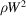, its pressure derivatives and the second- and third-order elastic constants  (p. 97) | html | pdf |